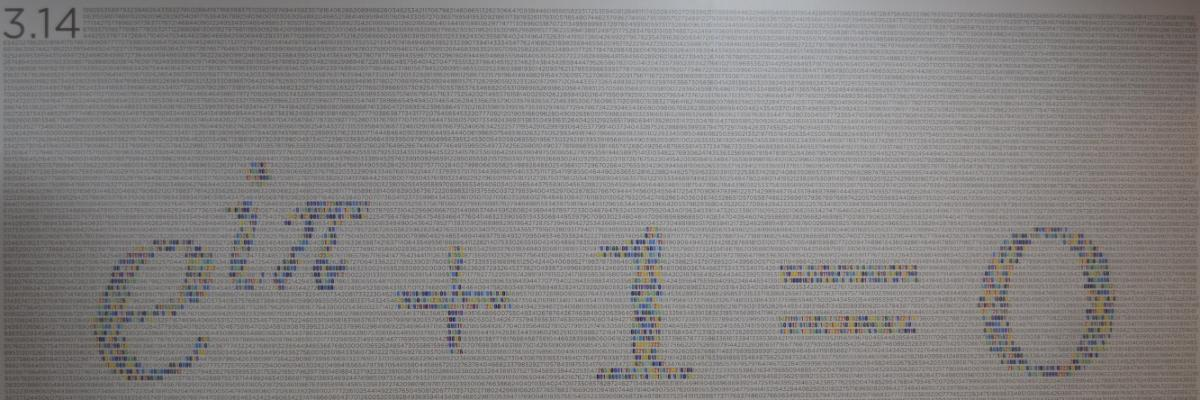RESEARCH GROUPThe main idea of the mathematics unit is to develop research that draws inspiration from and connects to different mathematical disciplines and also to encourage collaborations with scientists in areas such as biology, physics, social sciences, earth sciences, computer science, and many others, thus making it an integral part of the scientific ecosystem within ICTS.

The group pursues a wide range of mathematical, statistical and computational research themes. In particular, current research includes dynamical systems, probability, nonlinear partial differential equations, geometry and mathematical physics.

Main research areas:

• Algebraic Geometry:  Moduli spaces arising in quantum field theory and string theory, deformation theory, derived algebraic geometry, derived symplectic geometry, stability structures and extremal metrics, mirror symmetry, non-abelian Hodge theory and higher Teichmüller theory.
Faculty involved: Pranav Pandit

• Analysis of partial differential equations and applications: Analytical and numerical methods for PDEs. Boundary-value problems, stability of solutions to nonlinear PDEs, reduced and asymptotic models. Applications in surface gravity waves, geophysical fluid dynamics, monsoon modelling, inverse and observer problems for PDEs. Experimental fluid dynamics
Faculty involved: Vishal VasanJim Thomas

• Data assimilation: Bayesian data assimilation and inverse problems, consistency and convergence rates of posterior measures, Markov Chain Monte Carlo (MCMC) sampling methods, particle filtering, variational methods and observers for PDE models
Faculty involved: Amit Apte

• Differential geometry: Constant mean curvature surfaces, Minimal surfaces, Maximal surfaces in Lorentz-Minkowski space
Faculty involved: Rukmini Dey

• Dynamical systems: Hamiltonian systems, nontwist maps, bifurcations, transition to chaos and renormalization; Nonlinearity and non-Gaussianity in data assimilation and nonlinear filtering; Monsoon modelling
Faculty involved: Amit Apte

• Homotopical Mathematics: Higher category theory, higher algebraic structures, and their applications in mathematical physics; homotopy-theoretic and categorical methods in algebraic and symplectic geometry, derived noncommutative geometry, homological mirror symmetry.
Faculty involved: Pranav Pandit

• Mathematical physics: Quantization (especially geometric quantization) and coherent states; Geometric quantization of various moduli spaces of solutions of equations coming from physics; Quantization  (especially geometric quantization) of  Integrable systems, Study of moduli spaces of vortices, Higgs bundles, Generalized Seiberg-Witten equations etc.
Faculty involved: Rukmini Dey

• Monsoon dynamics: Conceptual models of monsoon dynamics, including ODE, PDE, and stochastic models; Data based studies of monsoon dynamics; Dynamics of cloud formation
Faculty involved: Amit Apte, Rama Govindarajan, Samriddhi Sankar Ray, Vishal Vasan

• Ocean dynamics: Mesoscale and Submesoscale flows, Turbulent energy transfer pathways, Internal gravity waves, Wave-Wave and Eddy-Wave interactions, Tracer dispersion and effective diffusivity, Asymptotic and Stochastic models for rotating and stratified turbulence.
Faculty involved:  Jim Thomas

• Probability Theory: First and last passage percolation;  Interacting particle system; Large Deviations; Random matrices and graphs; Statistical Physics
Faculty involved: Anirban Basak, Riddhipratim Basu

• Analysis of partial differential equations and their control aspects: Analytical methods to study the well-posedness of partial differential equations arising from fluid models and fluid-structure interaction models, their controllability, optimal control and stabilization.
Faculty involved : Mythily Ramaswamy

Activities (past and near future):

Opportunities:

The group envisions a significant and sustained growth in many areas and at all levels. Specific research areas that we would like to grow include, but are not limited to, probability and stochastic analysis, geometry and topology, mathematical physics, dynamical systems and differential equations, scientific computing, and discrete mathematics. ICTS would also like to develop a theoretical computer science group as part of this unit.